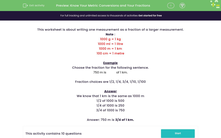### Comprehensive & curriculum aligned

In this worksheet, students convert a larger measurement to a smaller one, and then express the smaller one as a fraction of the larger one.Key stage:  KS 2

Curriculum topic:   Measurement

Curriculum subtopic:   Convert Between Metric Measurements

Difficulty level:#### Worksheet Overview

This worksheet is about writing one measurement as a fraction of a larger measurement.

Note :

1000 g = 1 kg

1000 ml = 1 litre

1000 m = 1 km

100 cm = 1 metre

Example

Choose the fraction for the following sentence.

750 m is  of 1 km.

Fraction choices are 1/2, 1/4, 3/4, 1/10, 1/100

We know that 1 km is the same as 1000 m

1/2 of 1000 is 500

1/4 of 1000 is 250

3/4 of 1000 is 750

Answer: 750 m is 3/4 of 1 km.

### What is EdPlace?

We're your National Curriculum aligned online education content provider helping each child succeed in English, maths and science from year 1 to GCSE. With an EdPlace account you’ll be able to track and measure progress, helping each child achieve their best. We build confidence and attainment by personalising each child’s learning at a level that suits them.

Get started